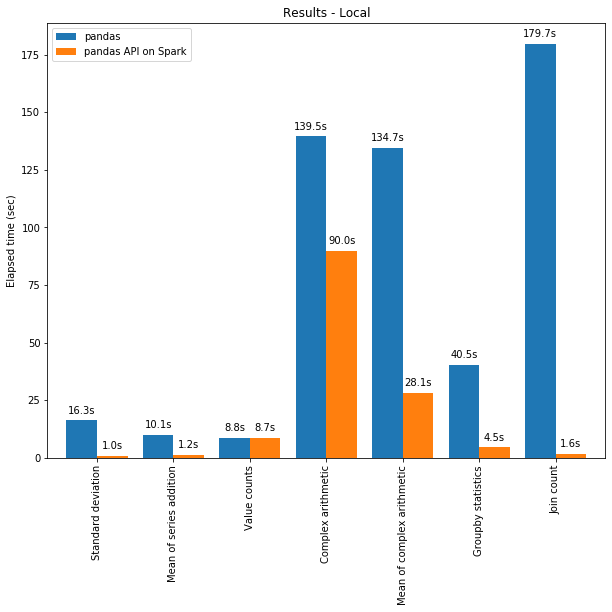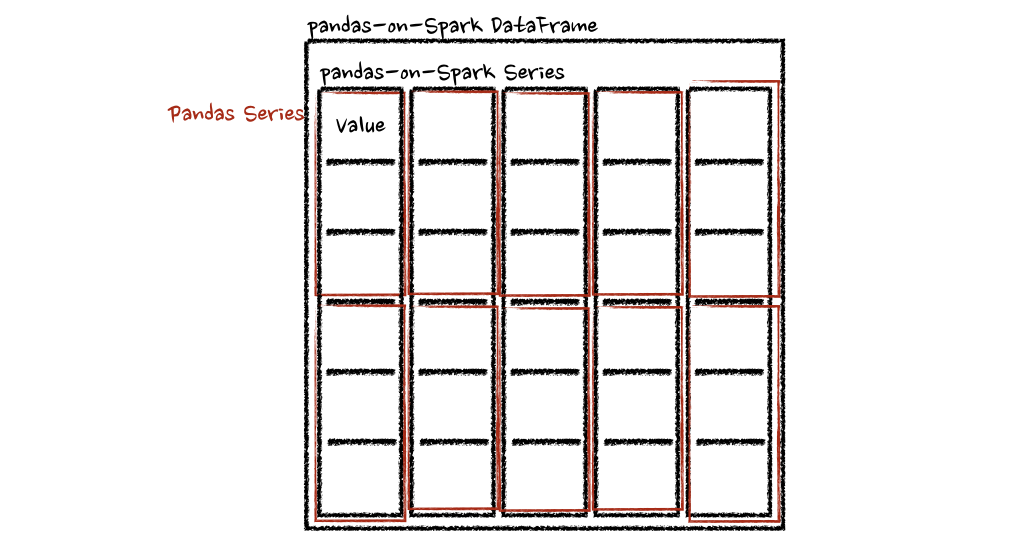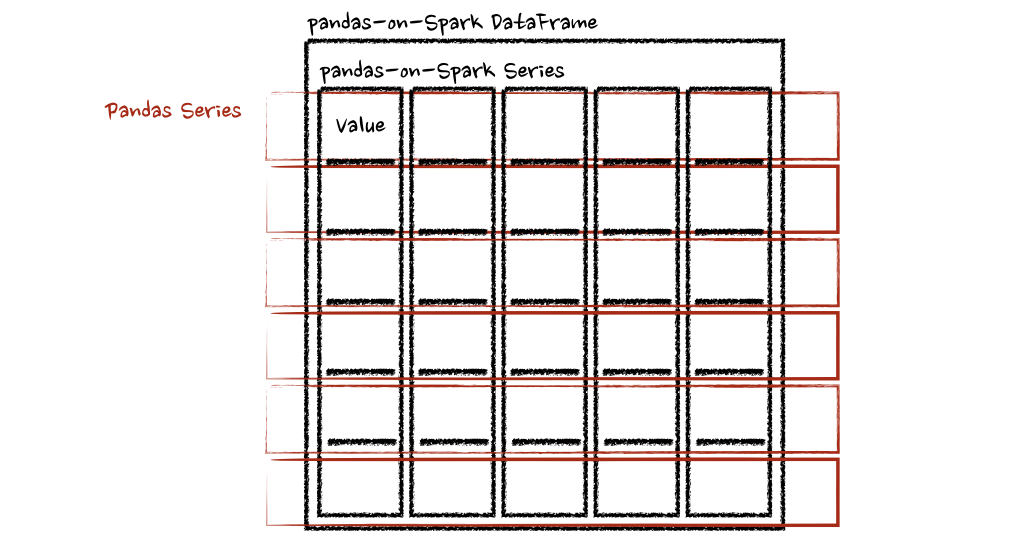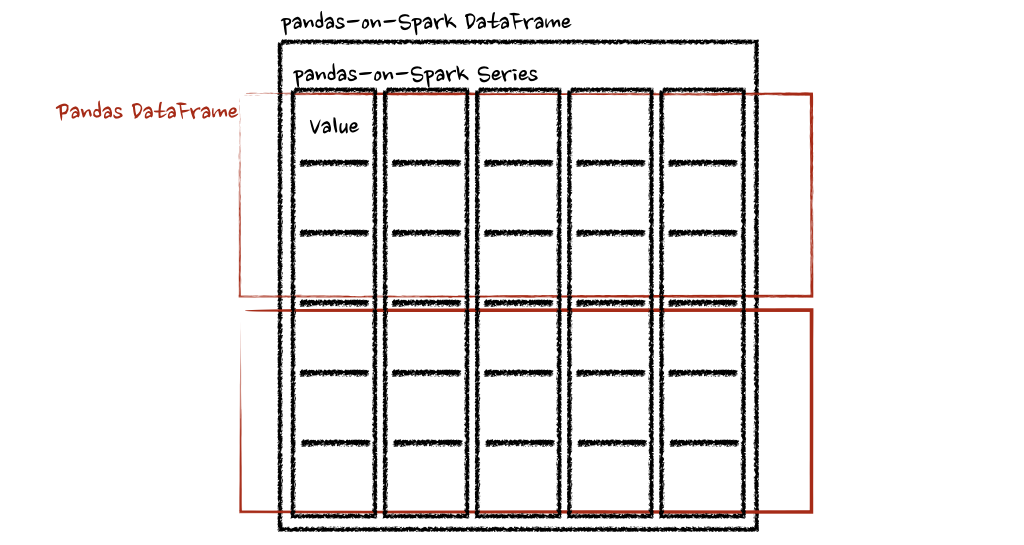# Pandas 与 PySpark 强强联手，功能与速度齐飞

Pandas做数据处理可以说是yyds！而它的缺点也是非常明显，Pandas 只能单机处理，它不能随数据量线性伸缩。例如，如果 pandas 试图读取的数据集大于一台机器的可用内存，则会因内存不足而失败。

Spark 现在集成了 Pandas API，因此可以在 Spark 上运行 Pandas。只需要更改一行代码：

``````import pyspark.pandas as ps
``````

• 如果我们熟悉使用Python 和 Pandas，但不熟悉 Spark，可以省略了需复杂的学习过程而立即使用PySpark。

• 可以为所有内容使用一个代码库：无论是小数据和大数据，还是单机和分布式机器。

• 可以在Spark分布式框架上，更快地运行 Pandas 代码。

### 技术提升Spark 在链式操作（chaining operations）中具有特别显着的优势。Catalyst 查询优化器可以识别过滤器以明智地过滤数据并可以应用基于磁盘的连接（disk-based joins），而 Pandas 倾向于每一步将所有数据加载到内存中。

## 在 Pandas / Pandas-on-Spark / Spark 之间切换

``````# import Pandas-on-Spark
import pyspark.pandas as ps

# 使用 Pandas-on-Spark 创建一个 DataFrame
ps_df = ps.DataFrame(range(10))

# 将 Pandas-on-Spark Dataframe 转换为 Pandas Dataframe
pd_df = ps_df.to_pandas()

# 将 Pandas Dataframe 转换为 Pandas-on-Spark Dataframe
ps_df = ps.from_pandas(pd_df)

``````

``````# 使用 Pandas-on-Spark 创建一个 DataFrame
ps_df = ps.DataFrame(range(10))

# 将 Pandas-on-Spark Dataframe 转换为 Spark Dataframe
spark_df = ps_df.to_spark()

# 将 Spark Dataframe 转换为 Pandas-on-Spark Dataframe
ps_df_new = spark_df.to_pandas_on_spark()

``````

## 数据类型如何改变？

``````>>> sdf = spark.createDataFrame([
...     (1, Decimal(1.0), 1., 1., 1, 1, 1, datetime(2020, 10, 27), "1", True, datetime(2020, 10, 27)),
... ], 'tinyint tinyint, decimal decimal, float float, double double, integer integer, long long, short short, timestamp timestamp, string string, boolean boolean, date date')
>>> sdf
``````
``````DataFrame[tinyint: tinyint, decimal: decimal(10,0),
float: float, double: double, integer: int,
long: bigint, short: smallint, timestamp: timestamp,
string: string, boolean: boolean, date: date]
``````
``````psdf = sdf.pandas_api()
psdf.dtypes
``````
``````tinyint                int8
decimal              object
float               float32
double              float64
integer               int32
long                  int64
short                 int16
timestamp    datetime64[ns]
string               object
boolean                bool
date                 object
dtype: object
``````

## Pandas-on-Spark vs Spark 函数

### 导入库

``````# 运行Spark
from pyspark.sql import SparkSession
spark = SparkSession.builder \
.appName("Spark") \
.getOrCreate()
# 在Spark上运行Pandas
import pyspark.pandas as ps
``````

### 读取数据

``````# SPARK
# PANDAS-ON-SPARK
``````

### 选择

``````# SPARK
sdf.select("sepal_length","sepal_width").show()
# PANDAS-ON-SPARK
``````

### 删除列

``````# SPARK
sdf.drop('sepal_length').show()# PANDAS-ON-SPARK
``````

### 删除重复项

``````# SPARK
sdf.dropDuplicates(["sepal_length","sepal_width"]).show()
# PANDAS-ON-SPARK
pdf[["sepal_length", "sepal_width"]].drop_duplicates()
``````

### 筛选

``````# SPARK
sdf.filter( (sdf.flower_type == "Iris-setosa") & (sdf.petal_length > 1.5) ).show()
# PANDAS-ON-SPARK
pdf.loc[ (pdf.flower_type == "Iris-setosa") & (pdf.petal_length > 1.5) ].head()
``````

### 计数

``````# SPARK
sdf.filter(sdf.flower_type == "Iris-virginica").count()
# PANDAS-ON-SPARK
pdf.loc[pdf.flower_type == "Iris-virginica"].count()
``````

### 唯一值

``````# SPARK
sdf.select("flower_type").distinct().show()
# PANDAS-ON-SPARK
pdf["flower_type"].unique()
``````

### 排序

``````# SPARK
sdf.sort("sepal_length", "sepal_width").show()
# PANDAS-ON-SPARK
``````

### 分组

``````# SPARK
sdf.groupBy("flower_type").count().show()
# PANDAS-ON-SPARK
pdf.groupby("flower_type").count()
``````

### 替换

``````# SPARK
sdf.replace("Iris-setosa", "setosa").show()
# PANDAS-ON-SPARK
``````

### 连接

``````#SPARK
sdf.union(sdf)
# PANDAS-ON-SPARK
pdf.append(pdf)
``````

## transform 和 apply 函数应用

``````DataFrame.transform()
DataFrame.apply()
DataFrame.pandas_on_spark.transform_batch()
DataFrame.pandas_on_spark.apply_batch()
Series.pandas_on_spark.transform_batch()
``````

### transform 和 apply

`DataFrame.transform()``DataFrame.apply()`之间的主要区别在于，前者需要返回相同长度的输入，而后者不需要。

``````# transform
psdf = ps.DataFrame({'a': [1,2,3], 'b':[4,5,6]})
def pandas_plus(pser):
return pser + 1  # 应该总是返回与输入相同的长度。

psdf.transform(pandas_plus)

# apply
psdf = ps.DataFrame({'a': [1,2,3], 'b':[5,6,7]})
def pandas_plus(pser):
return pser[pser % 2 == 1]  # 允许任意长度

psdf.apply(pandas_plus)
````````````psdf = ps.DataFrame({'a': [1,2,3], 'b':[4,5,6]})
def pandas_plus(pser):
return sum(pser)  # 允许任意长度
psdf.apply(pandas_plus, axis='columns')
``````#### `pandas_on_spark.transform_batch`和`pandas_on_spark.apply_batch`

batch 后缀表示 pandas-on-Spark DataFrame 或 Series 中的每个块。API 对 pandas-on-Spark DataFrame 或 Series 进行切片，然后以 pandas DataFrame 或 Series 作为输入和输出应用给定函数。请参阅以下示例：

``````psdf = ps.DataFrame({'a': [1,2,3], 'b':[4,5,6]})
def pandas_plus(pdf):
return pdf + 1  # 应该总是返回与输入相同的长度。

psdf.pandas_on_spark.transform_batch(pandas_plus)

psdf = ps.DataFrame({'a': [1,2,3], 'b':[4,5,6]})
def pandas_plus(pdf):
return pdf[pdf.a > 1]  # 允许任意长度

psdf.pandas_on_spark.apply_batch(pandas_plus)
``````## 在 Spark 上使用 pandas API的注意事项

### 不要使用重复的列名

``````import pyspark.pandas as ps
psdf = ps.DataFrame({'a': [1, 2], 'b':[3, 4]})
psdf.columns = ["a", "a"]
``````
``````Reference 'a' is ambiguous, could be: a, a.;
``````

``````import pyspark.pandas as ps
psdf = ps.DataFrame({'a': [1, 2], 'A':[3, 4]})
``````
``````Reference 'a' is ambiguous, could be: a, a.;
``````

``````from pyspark.sql import SparkSession
builder = SparkSession.builder.appName("pandas-on-spark")
builder = builder.config("spark.sql.caseSensitive", "true")
builder.getOrCreate()

import pyspark.pandas as ps
psdf = ps.DataFrame({'a': [1, 2], 'A':[3, 4]})
psdf
``````
``````   a  A
0  1  3
1  2  4
``````

### 使用默认索引

pandas-on-Spark 用户面临的一个常见问题是默认索引导致性能下降。当索引未知时，Spark 上的 Pandas API 会附加一个默认索引，例如 Spark DataFrame 直接转换为 pandas-on-Spark DataFrame。

### 在 Spark 上使用 pandas API

``````>>> import pandas as pd
>>> max(pd.Series([1, 2, 3]))
3
>>> min(pd.Series([1, 2, 3]))
1
>>> sum(pd.Series([1, 2, 3]))
6
``````

Pandas 数据集存在于单台机器中，自然可以在同一台机器内进行本地迭代。但是，pandas-on-Spark 数据集存在于多台机器上，并且它们是以分布式方式计算的。很难在本地迭代，很可能用户在不知情的情况下将整个数据收集到客户端。因此，最好坚持使用 pandas-on-Spark API。上面的例子可以转换如下：

``````>>> import pyspark.pandas as ps
>>> ps.Series([1, 2, 3]).max()
3
>>> ps.Series([1, 2, 3]).min()
1
>>> ps.Series([1, 2, 3]).sum()
6
``````

pandas 用户的另一个常见模式可能是依赖列表推导式或生成器表达式。但是，它还假设数据集在引擎盖下是本地可迭代的。因此，它可以在 pandas 中无缝运行，如下所示：

``````import pandas as pd
data = []
countries = ['London', 'New York', 'Helsinki']
pser = pd.Series([20., 21., 12.], index=countries)
for temperature in pser:
assert temperature > 0
if temperature > 1000:
temperature = None
data.append(temperature ** 2)

pd.Series(data, index=countries)
``````
``````London      400.0
New York    441.0
Helsinki    144.0
dtype: float64
``````

``````import pyspark.pandas as ps
import numpy as np
countries = ['London', 'New York', 'Helsinki']
psser = ps.Series([20., 21., 12.], index=countries)
def square(temperature) -> np.float64:
assert temperature > 0
if temperature > 1000:
temperature = None
return temperature ** 2

psser.apply(square)
``````
``````London      400.0
New York    441.0
Helsinki    144.0
``````

### 减少对不同 DataFrame 的操作

Spark 上的 Pandas API 默认不允许对不同 DataFrame（或 Series）进行操作，以防止昂贵的操作。只要有可能，就应该避免这种操作。

## 写在最后

### 参考资料

PySpark 指南: https://spark.apache.org/docs/latest/api/python/user_guide/pandas_on_spark/pandas_pyspark.html

PySpark 指南: https://spark.apache.org/docs/latest/api/python/user_guide/pandas_on_spark/types.html

默认索引类型: https://spark.apache.org/docs/latest/api/python/user_guide/pandas_on_spark/options.html#default-index-type